# 4.3. Running MCMC¶

This is the vanilla Monte Carlo Markov Chain with the Metropolis algorithm, as introduced in the previous section The Metropolis-Hastings sampler.

We will now proceed to run MCMC for the $$\Lambda\text{CDM}$$ model. The epic.py script accepts five commands (besides gui): run, analyze, plot, monitor and burst. The commands may be issued from any location if you are on Unix in an environment created with venv. In other configurations you may need to run the commands from the EPIC’s home directory and/or add python at the beginning of the command.

## Sampling the posterior distribution¶

Standard MCMC is the default option for sampling. We start a MCMC simulation with the run command:

$epic.py run /path/to/LCDM.ini 12 10000 --sim-full-name MyFirstRun --check-interval 6h  where the first argument is the .ini file, the second is the number of chains to be run in parallel and the third is the number of steps to be run in each MCMC iteration. This number of steps means that the chains will be written to the disk (the new states are appended to the chains files) after each steps states in all chains. A large number prevents frequent writing operations, which could impact overall performance unnecessarily. Each chain will create an independent process with Python’s multiprocessing, so you should not choose a number higher than the number of CPUs multiprocessing.cpu_count() of your machine. The number of chains should not be less than 2. If correlations in the initial proposal covariance matrix are needed or desired, the user can overwrite the diagonal proposal covariance matrix with the option --proposal-covariance followed by the name of the file containing the desired covariance matrix in a table format. The program creates a directory for this new simulation. Inside this directory, another one named chains receives the files that will store the states of the chains, named chain_0.txt, chain_1.txt, etc. Other relevant files are equally named but stored in the folder current_states. These store only the last state of each chain, to allow fast resuming from where the chain stopped, without need to loading the entire chains. All chains start at the same point, with coordinates given by the default values of each parameter, unless the option --multi-start is given, in which case they start at points randomly sampled from the priors. A good starting point may help to obtain convergence faster, besides eliminating the need for burn-in . The name of the folder is the date-time of creation (in UTC), unless the option --sim-full-name MyFirstRun is used, then MyFirstRun will be the folder name. A custom label or tag can also be prepended with the --sim-tag my_label option, for example, the folder my_label-171110-173000. It will be stored within another folder with the same name of the .ini file, thus in this case LCDM/MyFirstRun. The full path of the simulation will be displayed in the first line of the output. An existing simulation can be resumed from where it last saved information to disk with the same command, just giving the path of the simulation instead of the .ini file name, and omitting the number of chains, which has already been defined in the first run, for example: $ epic.py run <FULL-OR-RELATIVE-PATH-TO>/LCDM/MyFirstRun/ 5000


Another important parameter is the tolerance accepted for convergence assessment. By default, the program will stop when the convergence check finds, according to the Gelman-Rubin method, convergence with $$\epsilon < 0.01$$. To change this value, you can use --tolerance 0.002 or just -t 2e-3, for example. In the MCMC mode, the code will periodically check for convergence according to the Gelman-Rubin method (by default it is done every two hours but can be specified differently as --check-interval 12h or -c 45min in the arguments, for example. This does not need to (and should not) be a small time interval, but the option to specify this time in minutes or even in seconds (30sec) is implemented and available for testing purposes.

The relevant information will be displayed, in our example case looking similar to the following:

Simulation at <FULL-PATH-TO>/LCDM/MyFirstRun
Mode MCMC.
The following datasets will be used:
6dF+SDSS_MGS
HST_local_H0
JLA_simplified
Planck2015_distances_LCDM
SDSS_BOSS_CMASS
SDSS_BOSS_LOWZ
SDSS_BOSS_Lyalpha-Forests
SDSS_BOSS_QuasarLyman
SDSS_BOSS_consensus
cosmic_chronometers
Initiating MCMC...


and the MCMC will start.

The MCMC run stops if convergence is achieved with a tolerance smaller than the default or given tolerance.

The following is the output of our example after the MCMC has started. The 10000 steps take a bit more than thirty minutes in my workstation running 12 chains in parallel. The number of chains will not make much impact on this unless we use too many steps by iteration and work close to the machine’s memory limit. After approximately six hours, convergence is checked. Since it is larger than our required tolerance, the code continues with new iterations for another six hours before checking convergence again and so on. When convergence smaller than tolerance is achieved the code makes the relevant plots and quits.

Initiating MCMC...
i 1, 10000 steps, 12 ch; 32m45s, Sat Mar 24 00:29:13 2018. Next: ~5h27m.
i 2, 20000 steps, 12 ch; 32m53s, Sat Mar 24 01:02:07 2018. Next: ~4h54m.
i 3, 30000 steps, 12 ch; 32m52s, Sat Mar 24 01:34:59 2018. Next: ~4h21m.
i 4, 40000 steps, 12 ch; 32m54s, Sat Mar 24 02:07:54 2018. Next: ~3h48m.
i 5, 50000 steps, 12 ch; 32m51s, Sat Mar 24 02:40:46 2018. Next: ~3h15m.
i 6, 60000 steps, 12 ch; 32m53s, Sat Mar 24 03:13:39 2018. Next: ~2h42m.
i 7, 70000 steps, 12 ch; 33m3s, Sat Mar 24 03:46:43 2018. Next: ~2h9m.
i 8, 80000 steps, 12 ch; 32m52s, Sat Mar 24 04:19:35 2018. Next: ~1h36m.
i 9, 90000 steps, 12 ch; 32m55s, Sat Mar 24 04:52:30 2018. Next: ~1h3m.
i 10, 100000 steps, 12 ch; 32m52s, Sat Mar 24 05:25:23 2018. Next: ~31m3s.
i 11, 110000 steps, 12 ch; 32m46s, Sat Mar 24 05:58:10 2018. Checking now...
Monitoring convergence...                                    [##########]  100%
R-1 tendency: 7.993e-01, 8.185e-01, 7.745e-01
i 12, 120000 steps, 12 ch; 32m49s, Sat Mar 24 06:31:21 2018. Next: ~5h27m.
i 13, 130000 steps, 12 ch; 32m53s, Sat Mar 24 07:04:15 2018. Next: ~4h54m.
i 14, 140000 steps, 12 ch; 32m49s, Sat Mar 24 07:37:04 2018. Next: ~4h21m.
...


After the first loop, the user can inspect the acceptance ratio of the chains, updated after every loop in the section acceptance rates of the simulation_info.ini file. Chains presenting bad performance based on this acceptance rate will be discarded. The values considered as good performance are any rate in the interval from $$0.1$$ to $$0.5$$. This can be changed with --acceptance-limits 0.2 0.5, for example. If you want to completely avoid chain removal use --acceptance-limits 0 1.

When starting a new run, use the option --adapt FREE [ADAPT [STEPS]], where FREE is an integer representing the number of loops of free random-walk before adaptation, ADAPT is the actual number of loops during which adaptation will take place, and STEPS is the number of steps for both, pre-adapting and adapting phases. Note that the last two are optional. If STEPS is omitted, the number of steps of the regular loops will be used (from the mandatory steps argument); if only one argument is given, it is assigned to both FREE and ADAPT. At least one loop of free random-walk is necessary, so the starting states can serve as input for the adaptation phase. The total number of states in the two phases will be registered in the simulation_info.ini file (adaptive burn-in) as the minimal necessary burn-in size if one wants to keep the chains Markovian. The adaptation is an iterative process that updates the covariance matrix $$\mathbf{S}_t$$ of the proposal function for the current $$t$$-th state based on the chains history and goes as follows. Let $$\mathbf{\Sigma}_t$$ be a matrix derived from $$\mathbf{S}_t$$, defined by $$\mathbf{\Sigma}_t \equiv \mathbf{S}_t \, m/2.38^2$$, where $$m$$ is the number of free parameters. After the initial free random-walk phase, we calculate, for a given chain, the chain mean

$\mathbf{\bar{x}}_t = \frac{1}{t} \sum_{i=1}^{t} \mathbf{x}_i,$

where $$\mathbf{x}_i = \left(x_{1,i}, \ldots, x_{m,i}\right)$$ is the $$i$$-th state vector of the chain. The covariance matrix for the sampling of the next state $$t+1$$ will then be given by

$\mathbf{S}_{t+1} = e^{2 \theta_{t+1}} \mathbf{\Sigma}_{t+1},$

where $$\theta_{t+1} = \theta_{t} + \gamma_t \left( \eta_t - 0.234 \right)$$, $$\gamma_t = t^{-\alpha}$$, $$\theta_t$$ is a parameter that we set to zero initially, $$\alpha$$ is a number between $$0.5$$ and $$1$$, here set to $$0.6$$, $$\eta_t$$ is the current acceptance rate, targeted at $$0.234$$, and

$\mathbf{\Sigma}_{t+1} = \left(1 - \gamma_t \right) \mathbf{\Sigma}_t + \gamma_t \, \left(\mathbf{x}_t - \mathbf{\bar{x}}_t\right)^T \left(\mathbf{x}_t - \mathbf{\bar{x}}_t\right).$

In this program, the covariance matrix is updated based on the first chain and applied to all chains. This method is mostly based on the adaptation applied to the parallel tempering algorithm by Łącki & Miasojedow (2016) . The idea is to obtain a covariance matrix such that the asymptotic acceptance rate is $$0.234$$, considered to be a good value. Note, however, that this feature is in beta and may require some trial and error with the choice of both free random-walk and adaptation phases lengths. It might take too long for the proposal covariance matrix to converge and the simulation is not guaranteed to keep good acceptance rates with the final matrix obtained after the adaptation phase.

## Analyzing the chains¶

Once convergence is achieved and MCMC is finished, the code reads the chains and extract the information for the parameter inference. But this can be done to assess the results while MCMC is still going on. The distributions are compiled for a nice plot with the command analyze:

$epic.py analyze <FULL-OR-RELATIVE-PATH-TO>/LCDM/MyFirstRun/  Histograms are generated for the marginalized distributions using 20 bins or any other number given with --bins or -b. At any time one can run this command, optionally with --convergence or -c to check the state of convergence. By default, this will calculate $$\hat R^p - 1$$ for twenty different sizes (which you can change with --GR-steps) considering the size of the chains to provide an idea of the evolution of the convergence. Convergence is assessed based on the Gelman-Rubin criterium. I will not enter into details of the method here, but I refer the reader to the original papers   for more information. The variation of the original method for multivariate distribution is implemented. When using MCMC, all the chains are checked for convergence and the final resulting distribution which is analyzed and plotted is the concatenation of all the chains, since they are essentially all the same once they have converged. ### Additional options¶ If for some reason you want to view the results for an intermediate point of the simulation, you can tell the script to --stop-at 18000, everything will be analyzed until that point. If you want to check the random walk of the chains you can plot the sequences with --plot-sequences. This will make a grid plot containing all the chains and all parameters. Keep in mind that this can take some time and generate a big output file if the chains are very long. You can contour this problem by thinning the distributions by some factor --thin 10, for example. This also applies for the calculation of the correlation of the parameters in each chain, enabled with the --correlation-function option. We generally represent distributions by their histograms but sometimes we may prefer to exhibit smooth curves. Although it is possible to choose a higher number of histogram bins, this may not be sufficient and may required much more data. Much better (although possibly slow when there are too many states) are the kernel density estimates (KDE) tuned for Gaussian-like distributions . To obtain smoothed shapes use --kde. This will compute KDE curves for the marginalized parameter distributions and also the two-parameter joint posterior probability distributions. ## Making the triangle plots¶ The previous command will also produce the plots automatically (unless supressed with --dont-plot), but you can always redraw everything when you want, maybe you would like to tweak some colors? Loading the chains again is not necessary since the analysis already saves the information for the plots anyway. This is done with: $ epic.py plot <FULL-OR-RELATIVE-PATH-TO>/LCDM/MyFirstRun/


The code will find the longest chain analysis results and plot them. In this plot, you can, for example, choose the main color with --color m for magenta, or with any other Python color name, and omit the best-fit point mark with --no-best-fit-marks. You can always use any of C0, C1, …, C9, which refer to the colors in the current style’s palette. The default option is C0.

Plot ranges and eventually necessary factors of power of 10 are automatically handled by the code, unless you use --no-auto-range and --no-auto-factors or choose your own ranges or powers of 10 by specifying a dictionary with parameter label as keys and a pair of floats in a list or an integer power of 10 for the entries custom range and custom factors under the section analysis in the .ini file.

If you have used the option --kde previously in the analysis you need to specify it here too to make the corresponding plots, otherwise the histograms will be drawn.

The $$1\sigma$$ and $$2\sigma$$ confidence levels are shown and are written to the file hist_table.tex (and kde_table.tex if it is the case) inside the folder with the size of the chain preceeded by the letter n. These $$\LaTeX$$-ready files can be compiled to make a nice table in a PDF file or included in your paper as you want. To view and save information for more levels , use --levels 1 2 3 4 5.

You can further tweak your plots and tables. --use-tex will make the program render the plot using $$\LaTeX$$, fitting nicely to the rest of your paper. You can plot Gaussian fits together with the histograms (the Gaussian curve and the two first sigma levels), using --show-gaussian-fits, but probably only for the sake of comparison since this is not usually done in publications. --fmt can be used to set the number of figures to report the results (default is 5). There is --font-size to choose the size of the fonts, --show-hist to plot the histograms together with the smooth curves when --kde is used, --no-best-fit-marks to omit the indication of the coordinates of the best-fit point, --png to save images in png format besides the pdf files.

If you have nuisance parameters, you can opt to not show them with --exclude nuisance. This option can actually take the label of any parameter you choose not to include in the plot.

Going beyond the standard model and trying to detect a new parameter? After making kernel density estimates, you can calculate how many sigmas of detection with the option --detect xi, for example, where xi is the label of the parameter in the analysis. Note that this will only work if you also include the option --kde at this point.

Below we see the triangle plot of the histograms, with the default settings, in comparison with a perfected version using the smoothed distributions, the Python color C9, the $$\LaTeX$$ renderer, including the $$3\sigma$$ confidence level and excluding the nuisance parameter $$M$$.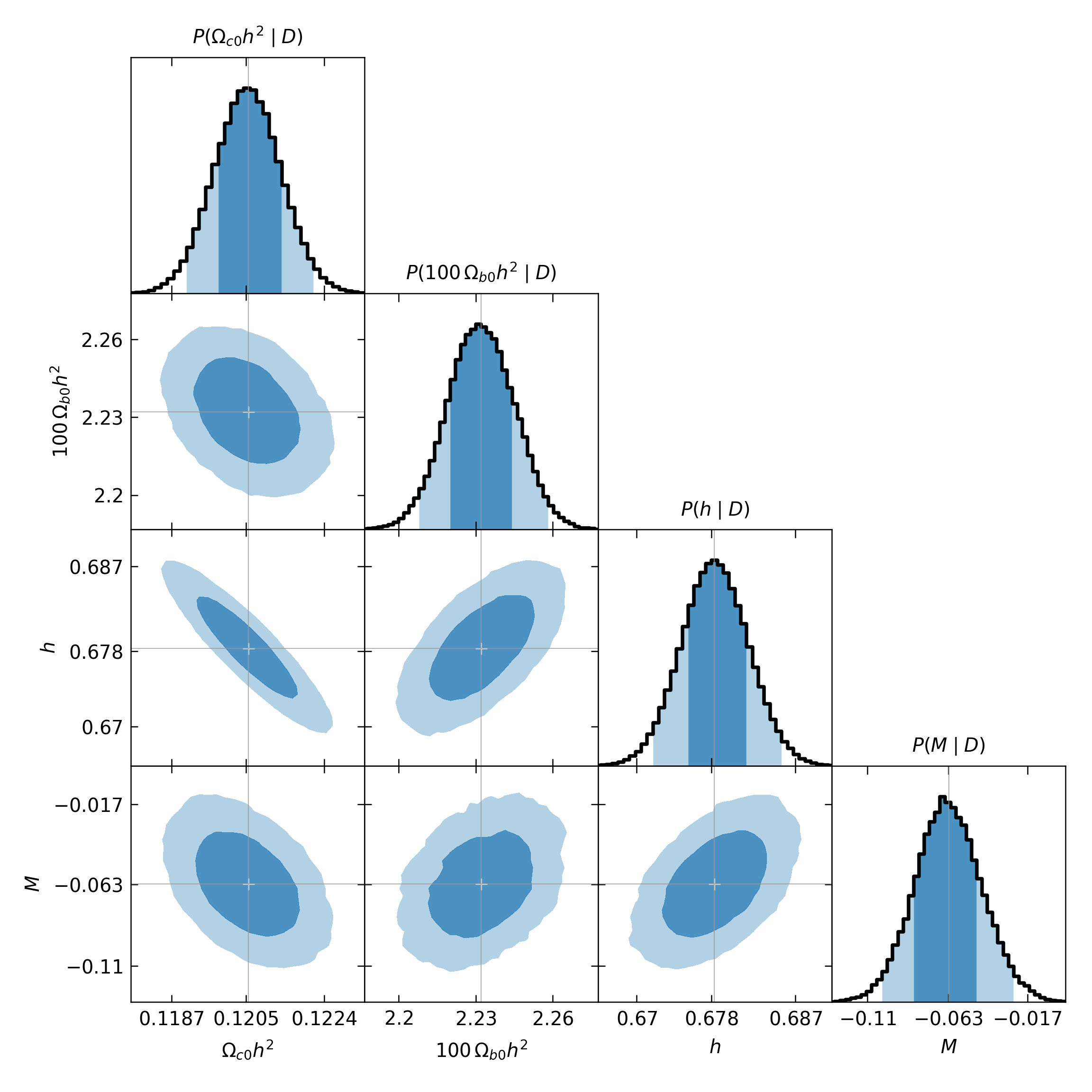Triangle plot with default configurations for histograms.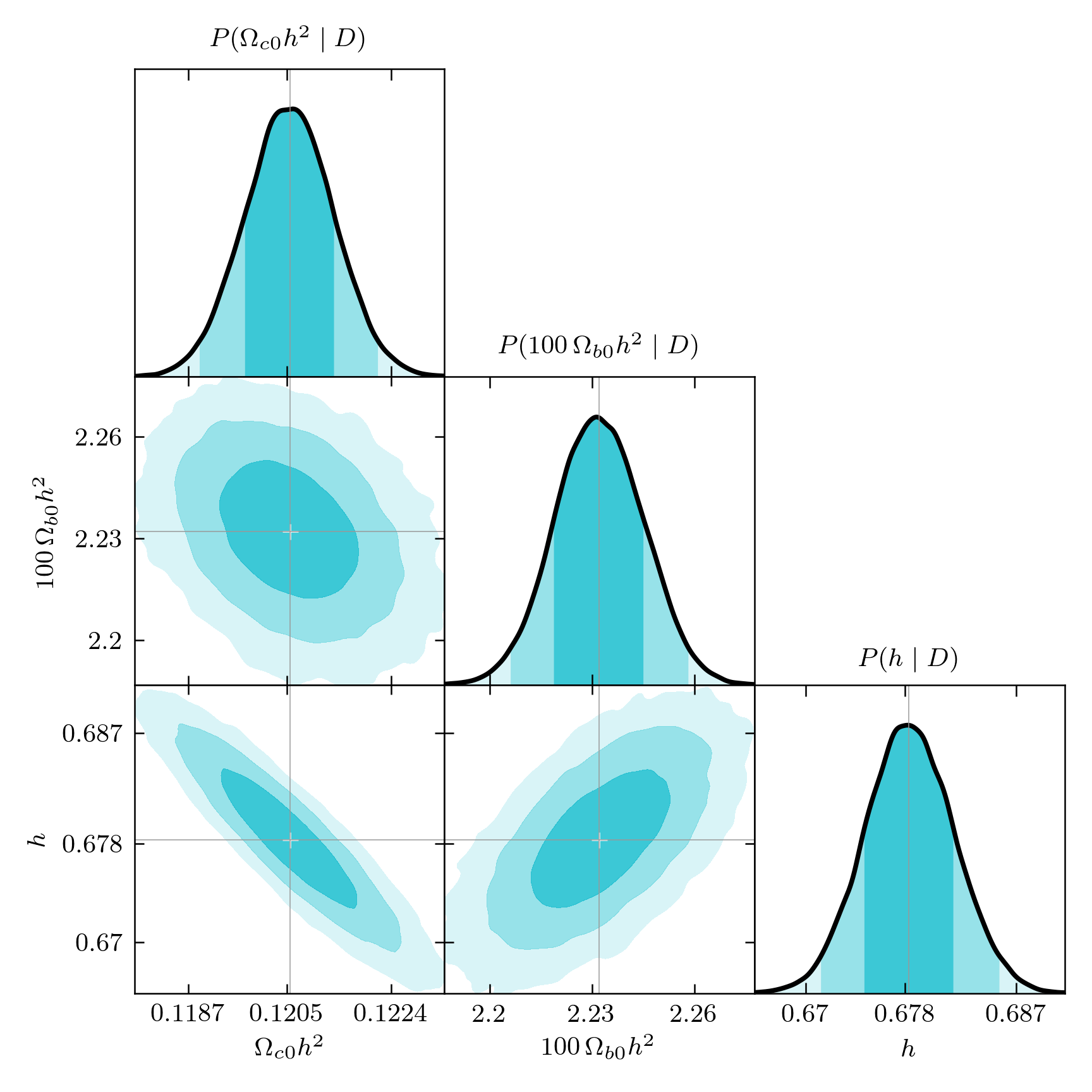Customized plot with smoothed distributions.

### Combining two or more simulations in one plot¶

You just need to run epic.py plot with two or more paths in the arguments. I illustrate this with two simulation for the same simplified $$\Lambda\text{CDM}$$ model, with cold dark matter and $$\Lambda$$ only, one with $$H(z)$$ and $$H_0$$ data, the other with the same data plus the simplified supernovae dataset from JLA. It is then interesting to plot both realizations together so we can see the effect that including a dataset has on the results:

$epic.py plot \ <FULL-OR-RELATIVE-PATH-TO>/HLCDM+SNeIa/H_and_SN/ \ <FULL-OR-RELATIVE-PATH-TO>/HLCDM/H_only/ \ --kde --use-tex --plot-prefix comparison --no-best-fit-marks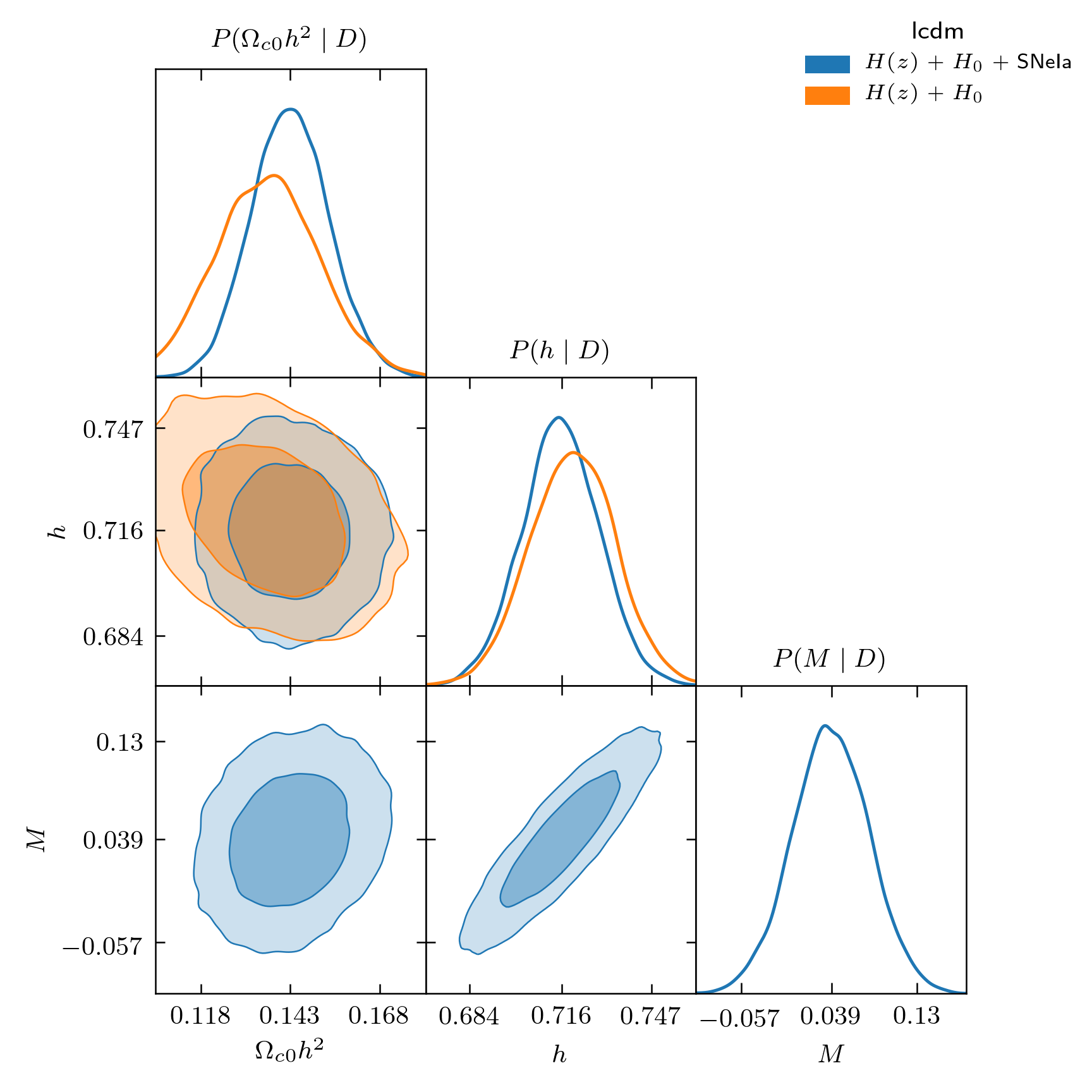Results for two different analyses. You can combine as many results as you like. When this is done, the list of parameters will be determined from the first simulation given in the command by its path. Automatic ranges for the plots are determined from the constraints of the first simulation as well. Since different simulations might give considerably different results that could go outside of the ranges of one of them, consider using --no-auto-range or specifying custom intervals in the .ini file if needed. The --plot-prefix option specifies the prefix for the name of the pdf file that will be generated. If you omit this option, grids2s will be used. All other settings are optional. A legend will be included in the top right corner using the labels defined in the .ini files, under the analysis section. The legend title uses the model name of the first simulation in the arguments. This is intended for showing, at the same time, results from different datasets with the same model. When generating plots of different analyses combined, you can customize the appearance in two ways. The first one, is changing the --color-scheme option, which defaults to tableau, to either xkcd, css4 or base. These are color palettes from matplotlib._color_data. XKCD_COLORS and CSS4_COLORS are dictionaries containing 949 and 148 colors each one, respectively. Because they are not ordered dictionaries, each time you plot using them you will get a different color scheme with random colors, so have fun! The four options can be followed by options like -light, -dark, etc (run $ epic.py plot --help to see the full list). These options just filter the lists returning only the colors that have that characteristic in their names.

Another way to customize plots is to specify a style with --style. The available options are default, bmh, dark_background, ggplot, Solarize_Light2, seaborn-bright, seaborn-colorblind, seaborn-dark-palette, seaborn-muted, seaborn-pastel and seaborn-whitegrid. These styles change the line colors and some also redefine the page background, text labels, plot background, etc. Be aware, however, that the previous option --color-scheme will overwrite the line colors of the style, unless you leave it in the default tableau option.

### Visualizing chain sequences and convergence¶

If you include the option --sequences with the analyze command you will get a plot like this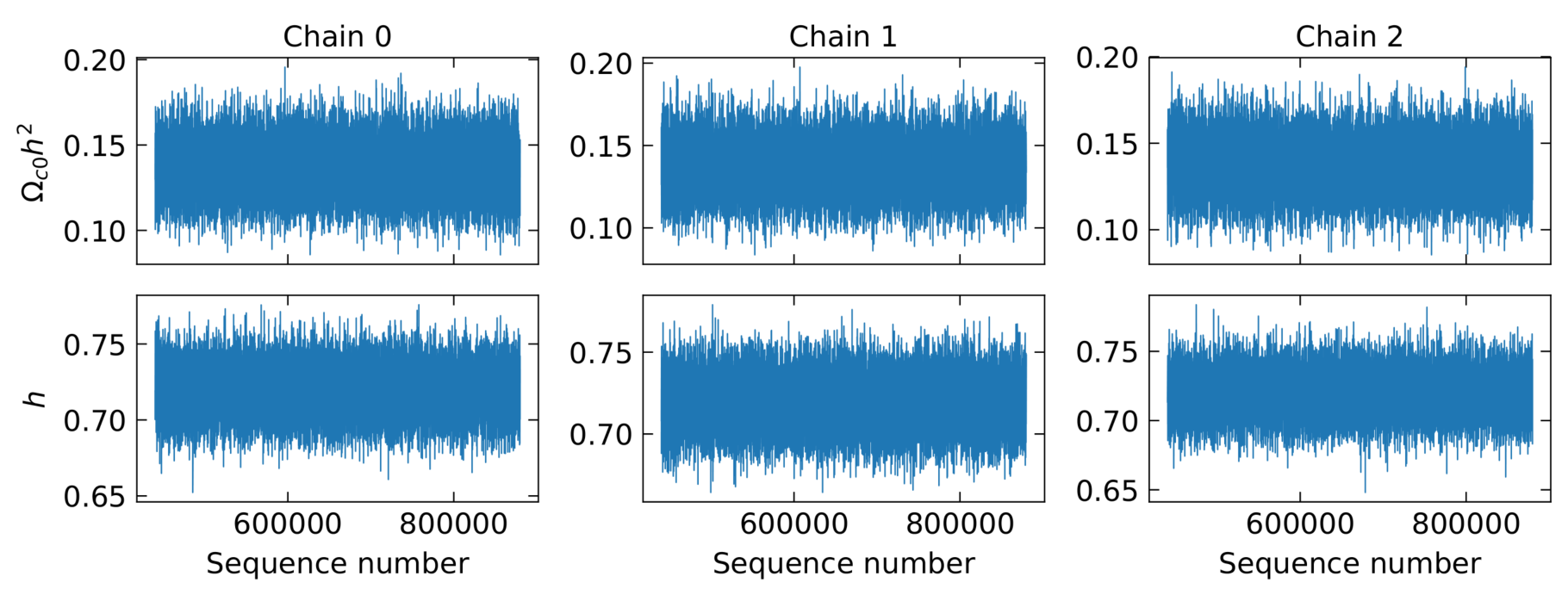Chain sequences along each parameter axis (only the first few chains shown here).

When monitoring convergence, the values of $$\hat{R}^p - 1$$ at twenty (or --GR-steps) different lengths for the multivariate analysis and separate one-dimensional analyses for each parameter are plotted in the files monitor_convergence_<N>.pdf and monitor_each_parameter_<N>.pdf, where N is the total utilized length of the chains. The absolute values of the between-chains and within-chains variances, $$\hat{V}$$ and $$W$$ are also shown. For our $$\Lambda\text{CDM}$$ example, we got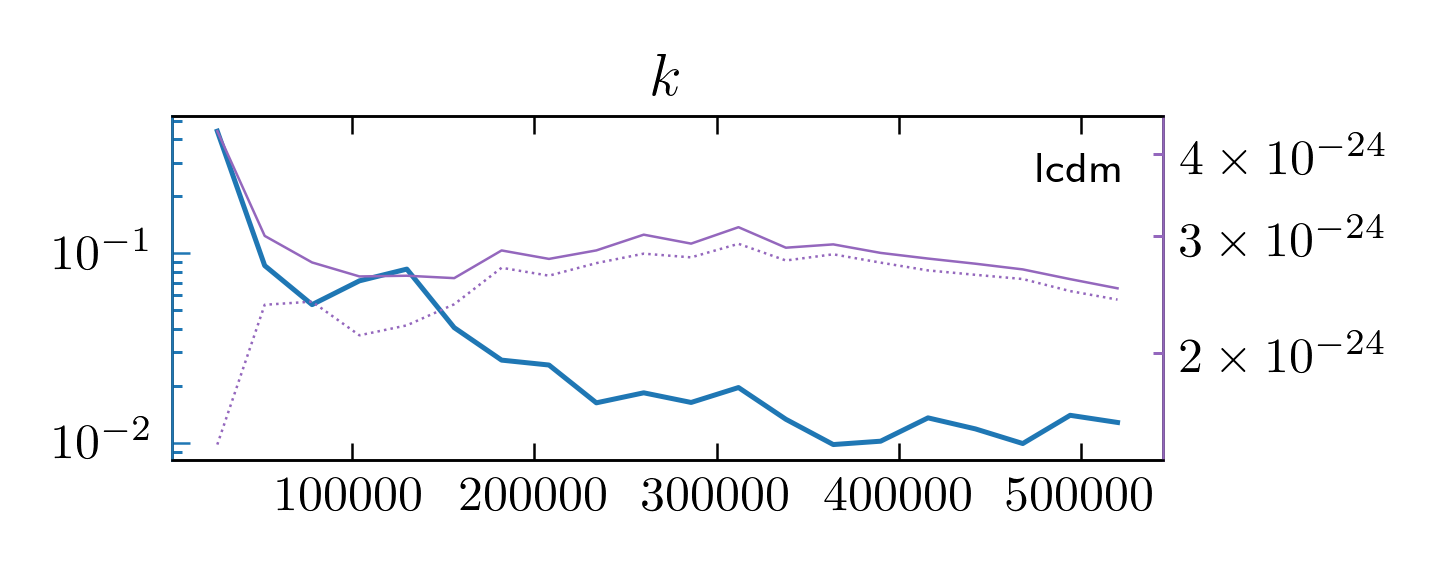Multivariate convergence analysis.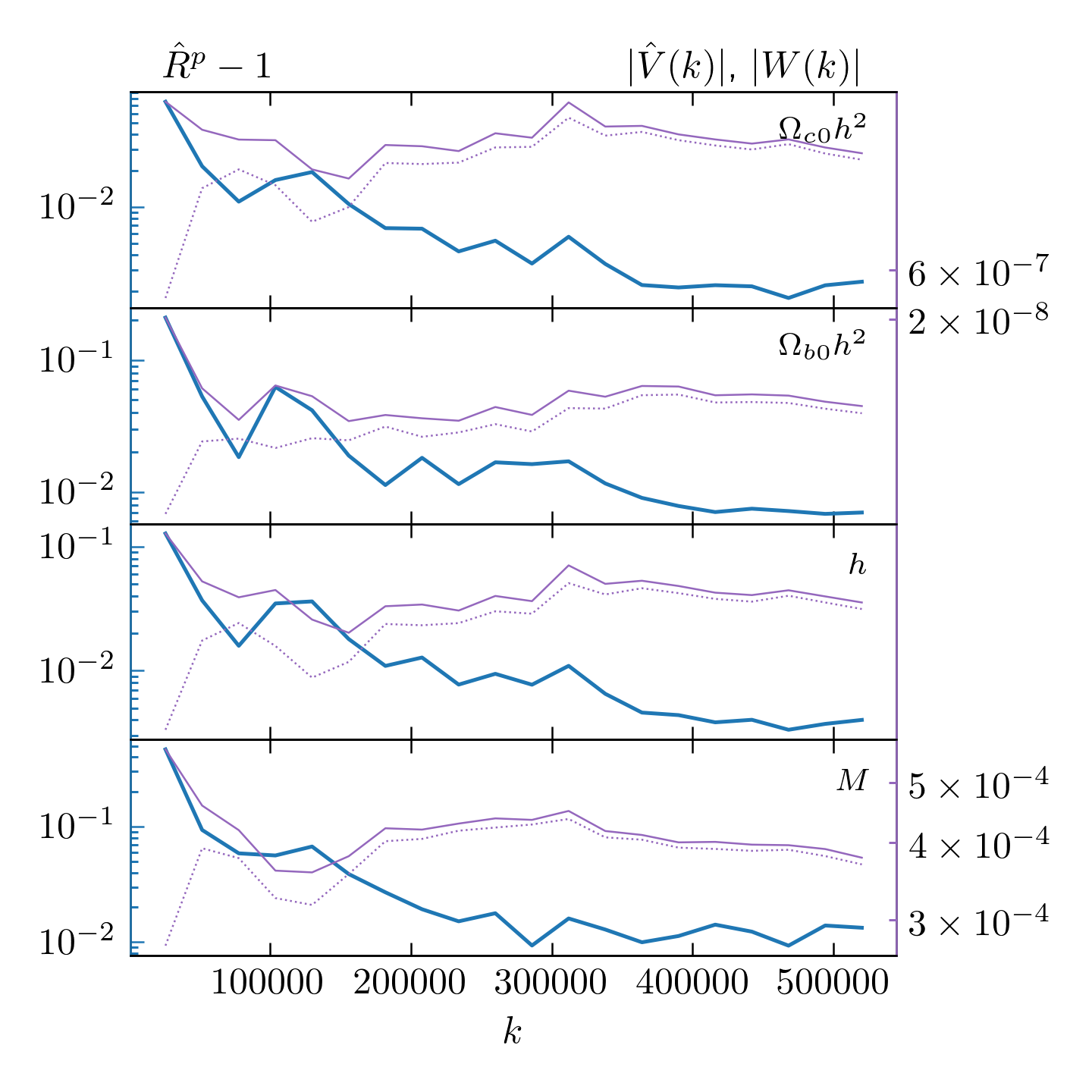Individual parameter convergence monitoring.

To generate these plots, one needs first run the script with the command analyze and the --convergence option; then, run:

$epic.py monitor <FULL-OR-RELATIVE-PATH-TO>/LCDM/MyFirstRun  The first argument can be multiple paths to simulations, in which case the first plot above will have panes for each simulation, one below each other. There are still the options --use-tex and --png, as with the plot command. Finally, the correlation of the chains can also be inspected if we use the option --correlation-function. This includes all the cross- and auto-correlations.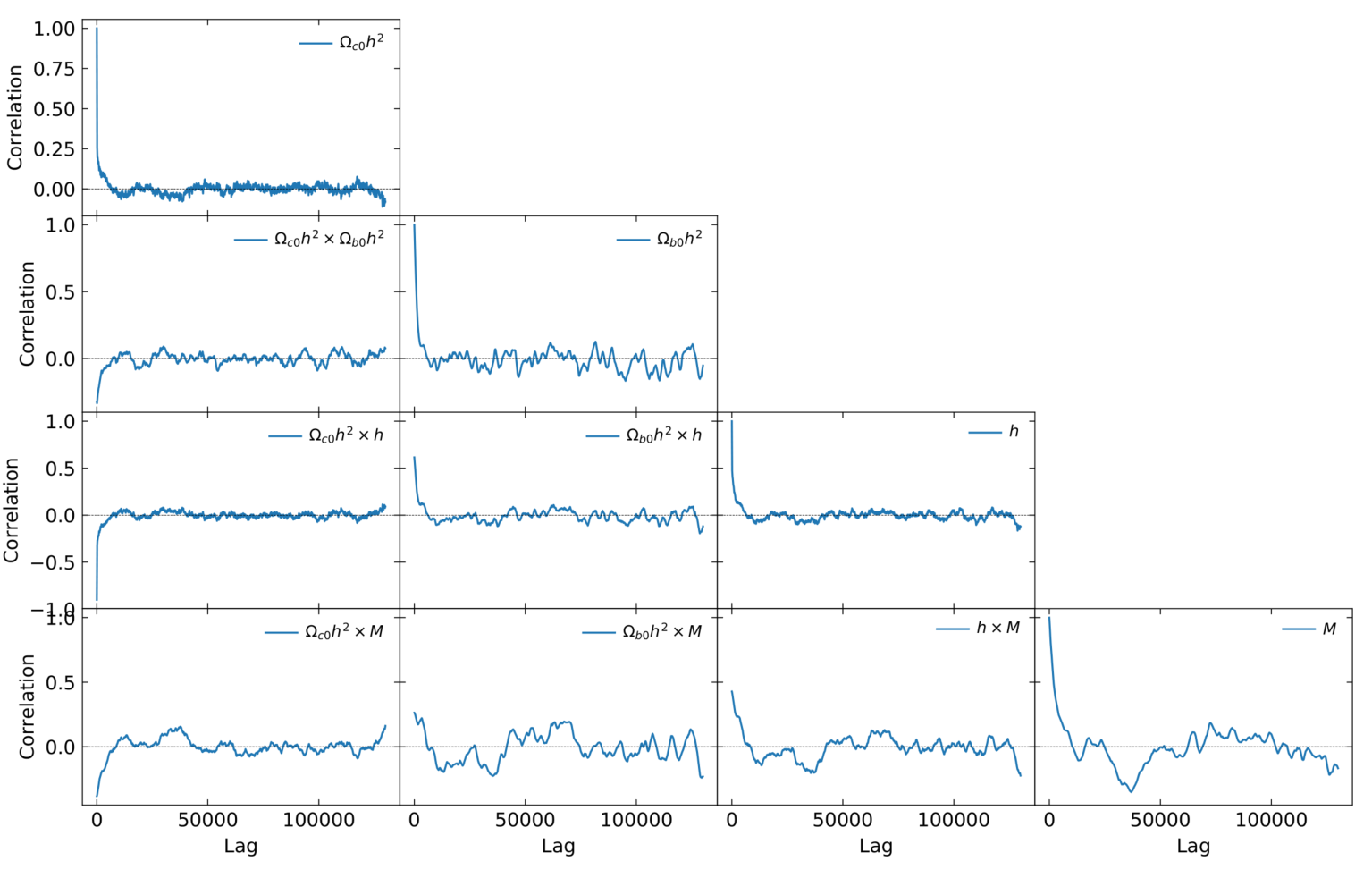Correlations in a chain (here using a factor --thin 15). ### Visualizing chains separately¶ With the command burst, the chains can be viewed separately, which may be useful to inspect unexpected behaviors of the distributions of the chains combined. Use: $ epic.py burst <FULL-OR-RELATIVE-PATH-TO>/<SIMULATION>


The script uses analyze for each chain separately, via the analyze’s option --use-chain and then plot with all the analyses performed. The option --use-chain is also available for burst, as well as the options --bins, --kde, --use-tex, --png and --plot-prefix. The figure bellow illustrates the result of this command.Triangle plot of separated chains from a simulation made with the command burst.

Footnotes

  When checking convergence with Gelman-Rubin method, however, burn-in is still applied.
  Łącki M. K. & Miasojedow B. “State-dependent swap strategies and automatic reduction of number of temperatures in adaptive parallel tempering algorithm”. Statistics and Computing 26, 951–964 (2016).
  Choice limited up to the fifth level. Notice that high sigma levels might be difficult to find due to the finite sample sizes when the sample is not sufficiently large.
  Gelman A & Rubin D. B. “Inference from Iterative Simulation Using Multiple Sequences”. Statistical Science 7 (1992) 457-472.
  Brooks S. P. & Gelman A. “General Methods for Monitoring Convergence of Iterative Simulations”. Journal of Computational and Graphical Statistics 7 (1998) 434.
  Kristan M., Leonardis A., Skocaj D. “Multivariate online kernel density estimation with Gaussian kernels”. Pattern Recognit 44 (2011) 2630-2642.